# 4 Digit Addition with Regrouping

4 Digit Addition with Regrouping  – Question 1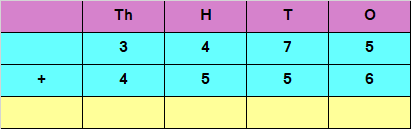Explanation

Adding the digits at ones place,
5 + 6 = 11
Since the number is more than 9, we carry over 1 to the tens place,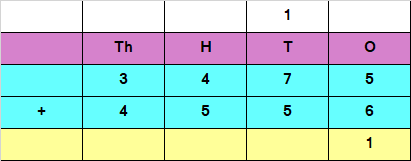Adding the digits at tens place and the carry of 1
7 + 5 + 1 = 13
Since the number is more than 9, we carry over 1 to the hundreds place,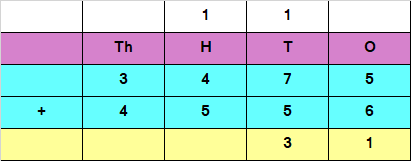Adding the digits at hundreds place and the carry of 1
1 + 4 + 5 = 10
Since the number is more than 9, we carry over 1 to the thousands place,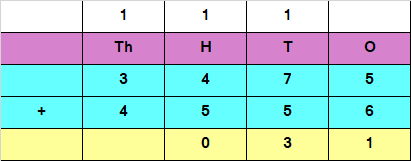Adding the digits at thousands place and the carry of 1
3 + 4 + 1 = 8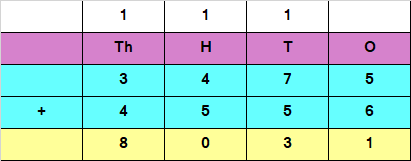Hence, the sum of the given numbers is 8031

4 Digit Addition with Regrouping  – Question 2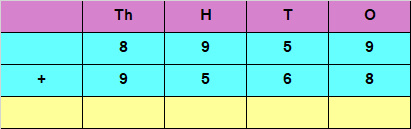Explanation

Adding the digits at ones place,
9 + 8 = 17
Since the number is more than 9, we carry over 1 to the tens place,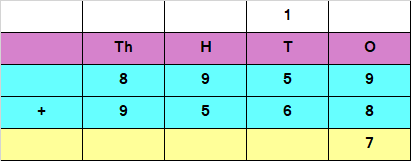Adding the digits at tens place and the carry of 1
5 + 6 + 1 = 12
Since the number is more than 9, we carry over 1 to the hundreds place,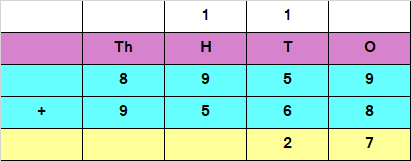Adding the digits at hundreds place and the carry of 1
9 + 5 + 1 = 15
Since the number is more than 9, we carry over 1 to the thousands place,Adding the digits at thousands place and the carry of 1
8 + 9 + 1 = 18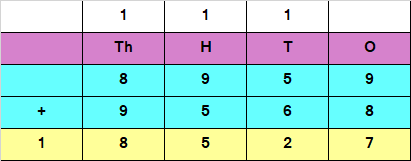Hence, the sum of the given numbers is 18527